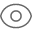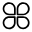# EF lambda表达式多条件查询984人阅读 2020/5/20 13:44 总访问：35770 评论:0 手机 收藏分类: Mvc EF框架

# lambda表达式多条件查询

clist = clist.Where(r => r.Price > 50 && r.Price < 60 || r.Price < 30).ToList();

List<car> clist= new cardata().SelectAll().Where(r => r.Name.Contains(TextBox1.Text)).ToList();

等于：==

clist = clist.Where(r => r.Price==40).ToList();

clist = clist.Where(r => r.Price.Equals(40)).ToList();

以。。开始、以。。结束

List<users> ulist = con.users.Where(r => r.username.StartsWith("s")).ToList();

List<users> ulsit = con.users.Where(r => r.username.EndsWith("t")).ToList();

int s = con.users.Max(r=>r.ids);

int t = con.users.Min(r=>r.ids);

double h = con.users.Average(r => r.ids);

int d = con.users.Sum(r => r.ids);

List<users> ulist1 = new List<users>();

List<users> ulist2 = new List<users>();

List<users> ulist3 = new List<users>();

List<users> ulist = ulist1.Intersect(ulist2).Intersect(ulist3).ToList();

List<users> ulist = con.users.OrderBy(r => r.ids).ToList();

List<users> ulist1 = con.users.OrderByDescending(r => r.ids).ToList();

{{item.content}}

## {{titleitem}} {{titleitem}}

{{item.content}}

{{item.articleTitle}}
{{item.blogName}} : {{item.content}}
ICP备案 ：渝ICP备18016597号-1